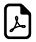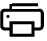Six Seven Eight Nine Times Tables - Hand Fingers Trick6 and 7 Times Tables - Fingers Trick(Applicable also 8 & 9)

STEP1: Hold your hands with palms facing you and fingers towards each other. Number the fingers on each hand from bottom to top as 6,7,8,9 and 10.STEP2: Multiplying 8 by 7, so the finger number 8 touches the finger number 7 on the other hand.STEP3: In the left hand, count the fingers above the touching finger =    3 fingers

On the same way in the right hand, count the fingers above the touching finger =    2 fingers

Multiply both = 3 x 2 = 6 ==>    1STEP4: In the left hand, count the finger which is touching 7 and the ones below that =    2 fingers

On the same way in the right hand, count the finger which is touching 8 and the ones below that =    3 fingers

Add the above counted fingers = 2 + 3 =    5 fingers

Multiply the number by 10 = 5 x 10 = 50 ==>    2STEP5: ==> Add    1    and    2   ,

= 50 + 6 = 56

Answer for 7 x 8 = 566,7,8 & 9 Times Multiplication Table Finger Tricks

An online 6 to 9 times table tricks.Easy way to learn the six to nine times table.Six to Nine Times Table LearningHow 6 to 9 times table works?

If you multiply 6 by an even number, they both end in the same digit.

Example:

6 x 2 = 12

6 x 4 = 24

6 x 6 = 36

Think 6, 7 , 8 and 9 times Table.Think Fingers !!!

What math times time do you want to generate ?Times Table Tricks 1 to 19999999
Multiply by 2 Digits Number Tricks
Tips & Tricks 1 to 15 Times Table
Multiply by any Number with Split Technique
Math Tricks for all Numbers with Build Down ApproachTop Calculators ►

Tax Calculator, Calories Burned Calculator, PERT, SD Calculator, Dog Age Calculator, Children Equal Playing Time Calculator